International
Tables for
Crystallography
Volume B
Reciprocal space
Edited by U. Shmueli

International Tables for Crystallography (2006). Vol. B. ch. 1.2, p. 11   | 1 | 2 |

## Section 1.2.5.2. Magnetic scattering

P. Coppensa*

aDepartment of Chemistry, Natural Sciences & Mathematics Complex, State University of New York at Buffalo, Buffalo, New York 14260-3000, USA
Correspondence e-mail: coppens@acsu.buffalo.edu

#### 1.2.5.2. Magnetic scattering

| top | pdf |

The interaction between the magnetic moments of the neutron and the unpaired electrons in solids leads to magnetic scattering. The total elastic scattering including both the nuclear and magnetic contributions is given by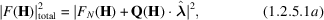where the unit vector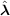describes the polarization vector for the neutron spin,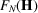is given by (1.2.4.2b)and Q is defined by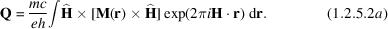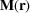is the vector field describing the electron-magnetization distribution and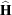is a unit vector parallel to H.

Q is thus proportional to the projection of M onto a direction orthogonal to H in the plane containing M and H. The magnitude of this projection depends on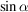, where α is the angle between Q and H, which prevents magnetic scattering from being a truly three-dimensional probe. If all momentsare collinear, as may be achieved in paramagnetic materials by applying an external field, and for the maximum signal (H orthogonal to M), (1.2.5.2a)becomes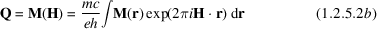and (1.2.5.1a)gives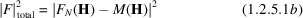and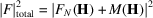for neutrons parallel and antiparallel to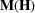, respectively.